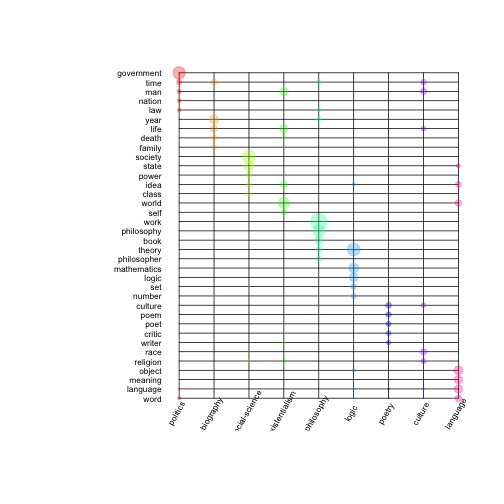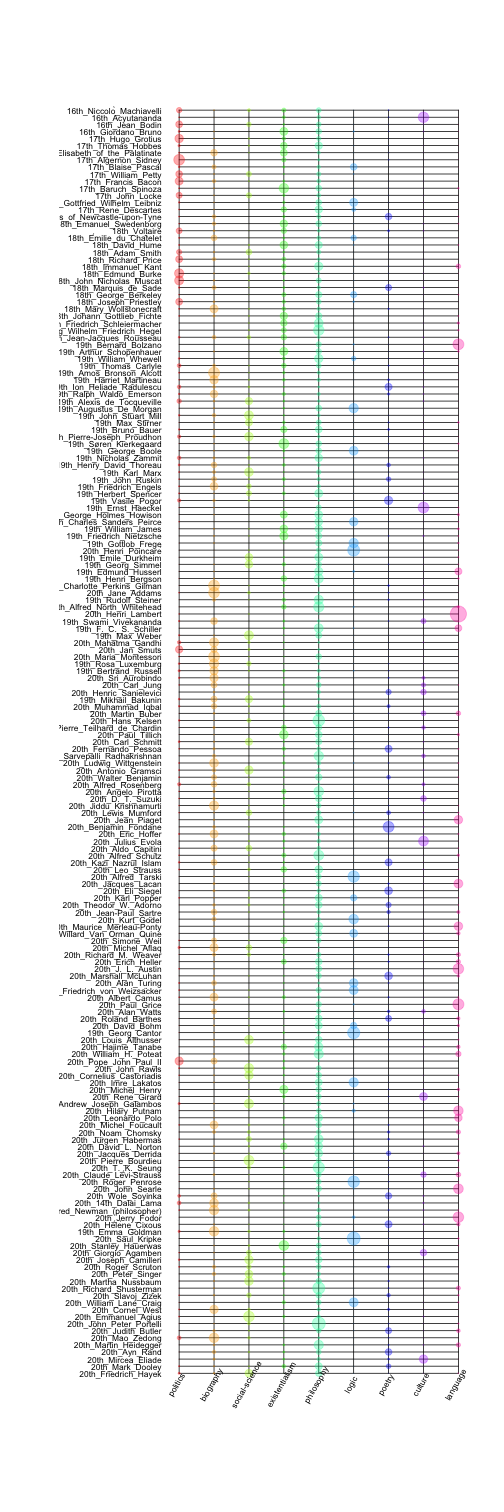# Topic Models

Patrick O. Perry, NYU Stern School of Business

Most of the code here is taken from Chapter 10.3 of Taylor Arnold and Lauren Tilton's book, Humanities Data in R.

## Preliminaries

### Computing environment

We will use the following R packages.

``````library("mallet")
library("coreNLP")
``````

To ensure consistent runs, we set the seed before performing any analysis.

``````set.seed(0)
``````

### Data

Arnold and Tilton have provided tagged versions of the wikipedia pages for 179 philosophers. The data is available on their webpage (humanitiesDataInR.zip).

``````wikiFiles <- dir("data/ch10/wiki_annotations", full.names=TRUE)
wikiNames <- gsub("\\.Rds", "", basename(wikiFiles))
``````

## Data preprocessing

### Order by year

The pre-processed corpus includes a `Timex` annotation for times. The following code pulls out the first year mentioned in each article; this is the philosopher's birth year.

``````dateSet <- rep(0, length(wikiFiles))
for (j in seq_along(wikiFiles)) {
tx <- getToken(anno)\$Timex
tx <- substr(tx[!is.na(tx)], 1, 4)
suppressWarnings({
tx <- as.numeric(tx)
})
tx <- tx[!is.na(tx)]
dateSet[j] <- tx
}
``````

Next, we order the documents by year

``````wikiFiles <- wikiFiles[order(dateSet)]
wikiNames <- wikiNames[order(dateSet)]
``````

### Feature selection

Rather than fitting the topic model to the entire text, we fit the model to just the lemmas of the non-proper nouns. The following code segment filters the text using the POS-tagged and lemmatized corpus. For each document, we build a long text string containing all of the selected words, separated by spaces.

``````bagOfWords <- rep("", length(wikiFiles))
for (j in seq_along(wikiFiles)) {
token <- getToken(anno)
theseLemma <- token\$lemma[token\$POS %in% c("NNS", "NN")]
bagOfWords[j] <- paste(theseLemma, collapse=" ")
}
``````

To filter out stopwords, we need to store the words in a file. Since we already have used POS tags to filter out stop words, we only need to worry about initials that may have been mistaken for non-proper nouns by the tagger.

``````tf <- tempfile()
writeLines(c(letters, LETTERS), tf)
``````

## Fitting

Here is code to train the topic model:

``````instance <- mallet.import(id.array=wikiNames, text.array=bagOfWords,
stoplist.file=tf)
tm <- MalletLDA(num.topics=9)
tm\$setAlphaOptimization(20, 50)
tm\$train(200)
tm\$maximize(10)
``````

These options specify how often to optimize the hyper-parameters (optimize alpha every 20 iterations after performing 50 burn-in iterations), how many training iterations to perform (200), and how iterations to use to determine the topics of each token (10). The values used here are the defaults suggested by the `mallet` package. Increasing these values may result in more consistent runs of the procedure.

After fitting the model we can now pull out the topics, the words, and the vocabulary:

``````topics <- mallet.doc.topics(tm, smoothed=TRUE, normalized=TRUE)
words <- mallet.topic.words(tm, smoothed=TRUE, normalized=TRUE)
vocab <- tm\$getVocabulary()
``````

Here are the dimensions of these objects:

``````dim(topics)
``````
`````` 179   9
``````
``````dim(words)
``````
``````     9 12123
``````
``````length(vocab)
``````
`````` 12123
``````

## Results

Here are the top 5 words in each of the 9 topics:

``````t(apply(words, 1, function(v) vocab[order(v, decreasing=TRUE)[1:5]]))
``````
``````      [,1]       [,2]          [,3]         [,4]          [,5]
[1,] "man"      "faith"       "death"      "religion"    "view"
[2,] "theory"   "society"     "view"       "development" "child"
[3,] "theory"   "mathematics" "logic"      "set"         "science"
[4,] "people"   "movement"    "party"      "war"         "leader"
[5,] "language" "book"        "culture"    "field"       "relationship"
[6,] "work"     "time"        "writer"     "poet"        "poem"
[7,] "year"     "family"      "father"     "life"        "school"
[8,] "law"      "state"       "government" "society"     "theory"
[9,] "work"     "philosophy"  "idea"       "world"       "life"
``````

The output of the topic model is sensitive to the random initialization. You will likely get different results every time you run this code. I do not know if it's possible to ensure consistent output from `mallet`. If you want your analysis to be reproducible, you should save the topic model output using a command like `saveRDS(list(topics=topics, words=words, vocab=vocab), "tm.rds")`.

For the remainder of this analysis, we will use the results from Arnold and Tilton's analysis:

``````tm <- readRDS("data/ch10/tm.Rds")
topics <- tm\$topics
words <- tm\$words
vocab <- tm\$vocab
t(apply(words, 1, function(v) vocab[order(v, decreasing=TRUE)[1:5]]))
``````
``````      [,1]         [,2]          [,3]       [,4]       [,5]
[1,] "government" "time"        "man"      "nation"   "law"
[2,] "year"       "life"        "time"     "death"    "family"
[3,] "society"    "state"       "power"    "idea"     "class"
[4,] "world"      "life"        "man"      "idea"     "self"
[5,] "work"       "philosophy"  "book"     "theory"   "philosopher"
[6,] "theory"     "mathematics" "logic"    "set"      "number"
[7,] "culture"    "poem"        "poet"     "critic"   "writer"
[8,] "man"        "race"        "time"     "religion" "life"
[9,] "object"     "meaning"     "language" "word"     "world"
``````

Commonly, people assign labels to the topics, based on the top 5 words.

``````topicNames <- c("politics", "biography", "social-science", "existentialism",
"philosophy", "logic", "poetry", "culture", "language")
``````

The next code segment shows the words with the highest activations for the topics:

``````index <- order(apply(words, 2, max), decreasing=TRUE)[1:50]
set <- unique(as.character(apply(words, 1, function(v)
vocab[order(v, decreasing=TRUE)][1:5])))
index <- match(set, vocab)
mat <- round(t(words[,index]), 3)
mat <- mat / max(mat)

plot(0, 0, col="white", t="n", axes=FALSE, xlab="", ylab="",
ylim=c(-1, nrow(mat)), xlim=c(-2,ncol(mat)))
for (i in seq_len(nrow(mat))) {
lines(x=c(1,ncol(mat)), y=c(i,i))
}
for (i in seq_len(ncol(mat))) {
lines(x=c(i,i), y=c(1,nrow(mat)))
}
points(col(mat), nrow(mat) - row(mat) + 1, pch=19,
cex=mat*3, col=rainbow(ncol(mat), alpha=0.33)[col(mat)])
text(1:ncol(mat), -0.75, topicNames, adj=c(0.5,0), cex=0.7, srt=60)
``````We can also plot the topic distributions of the documents in the corpus:

``````mat <- topics / max(topics)
plot(0, 0, col="white", t="n", axes=FALSE, xlab="", ylab="",
ylim=c(-1, nrow(mat)), xlim=c(-2,ncol(mat)))
for (i in seq_len(nrow(mat))) {
lines(x=c(1,ncol(mat)), y=c(i,i))
}
for (i in seq_len(ncol(mat))) {
lines(x=c(i,i), y=c(1,nrow(mat)))
}
points(col(mat), nrow(mat) - row(mat) + 1, pch=19,
cex=mat*3, col=rainbow(ncol(mat), alpha=0.33)[col(mat)])
text(1:ncol(mat), -0.75, topicNames, adj=c(0.5,0), cex=0.7, srt=60)
``````## Session information

``````sessionInfo()
``````
``````R version 3.2.3 (2015-12-10)
Platform: x86_64-apple-darwin13.4.0 (64-bit)
Running under: OS X 10.10.5 (Yosemite)

locale:
 en_US.UTF-8/en_US.UTF-8/en_US.UTF-8/C/en_US.UTF-8/en_US.UTF-8

attached base packages:
 methods   stats     graphics  grDevices utils     datasets  base

other attached packages:
 coreNLP_0.4-1      mallet_1.0         rJava_0.9-8        RColorBrewer_1.1-2 knitr_1.12.3

loaded via a namespace (and not attached):
 magrittr_1.5     formatR_1.1      tools_3.2.3      codetools_0.2-14 stringi_1.0-1
 plotrix_3.6-1    digest_0.6.8     stringr_1.0.0    XML_3.98-1.3     evaluate_0.8
``````